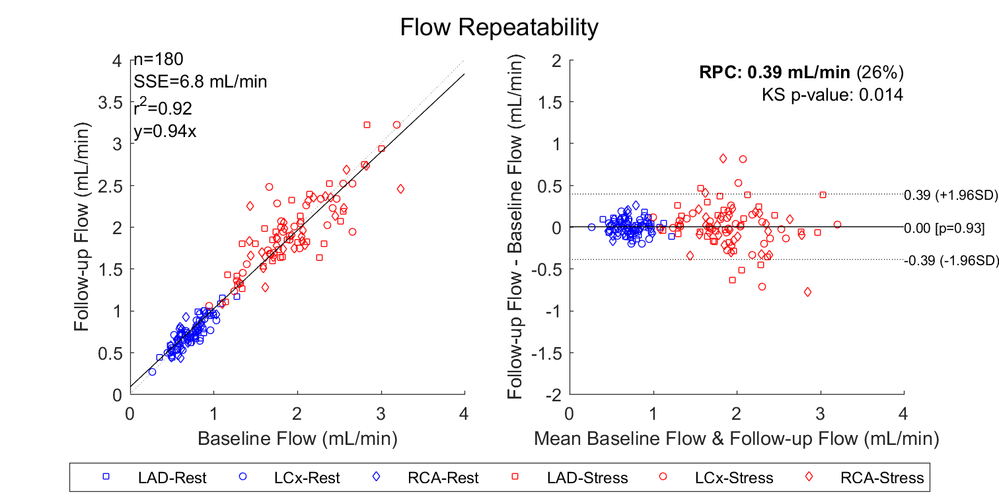## Reference Line of 0 centered for Proc SGPLOT (Bland-Altman)

This should be easy. I'm trying to make a Bland-Altman plot with a reference line at 0- but the reference line will show at the bottom, I need it centered on the y-axis.

proc sgplot data = EZ3;

refline 0 / axis =Y;

scatter y = difference x = average;

run;

quit;

In all examples I'm seeing online this is the (basic) way to do it and the reference line will be centered at 0 on the Y-axis with code like this.

For reference, I created a "fake" dataset that is exactly 3 times higher than the original dataset, so what I am looking at looks like a regression line since the 0 is not centered on the y-axis.

1 ACCEPTED SOLUTION

Accepted Solutions

## Re: Reference Line of 0 centered for Proc SGPLOT (Bland-Altman)

Add a yaxis statement with a value list. Something like

yaxis values= (-25 to 25 by 5);

or

yaxis values= (-10 -5 0 5 10);

with the range as suitable for your data. That should force an axis with the 0 in the middle

4 REPLIES 4

## Re: Reference Line of 0 centered for Proc SGPLOT (Bland-Altman)

I don't understand what you mean by 'centered at 0 on the Y axis'. Could you provide a sample or visual to help with this?

## Re: Reference Line of 0 centered for Proc SGPLOT (Bland-Altman)

Attached you will see the Bland-Altman plot on the right, you'll see 0 is centered on the Y-axis. Regression on the left for comparison.## Re: Reference Line of 0 centered for Proc SGPLOT (Bland-Altman)

Add a yaxis statement with a value list. Something like

yaxis values= (-25 to 25 by 5);

or

yaxis values= (-10 -5 0 5 10);

with the range as suitable for your data. That should force an axis with the 0 in the middle

## Re: Reference Line of 0 centered for Proc SGPLOT (Bland-Altman)

Thanks! Totally works.

Discussion stats
• 4 replies
• 837 views
• 2 likes
• 3 in conversation